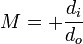# Optics question

If I have a camera orbiting in space way above the Earth, and it has a diffraction limited resolution of 4.1*10^-7 m (diameter of airy disc), then how can I find out the corresponding value if the lens is supposed to take images of the Earth?

mgb_phys
Homework Helper
What do you mean by "corresponding value"?
Presumably by the 4.1*10^-7 m you mean the resolution limit at the image plane.
If you knowthe focal length and orbital height you can work out the resolutionsize on the earth's surface

What do you mean by "corresponding value"?
Presumably by the 4.1*10^-7 m you mean the resolution limit at the image plane.
If you knowthe focal length and orbital height you can work out the resolutionsize on the earth's surface

Yeah, how do I figure out the res on the Earth? I know the Focal length and orbital height. I was thinking you could just multiply the airy disk size by 100000 for 100km

Redbelly98
Staff Emeritus
Homework Helper
Note that multiplying (airy disc diameter) x (orbital height) gives incorrect units of m^2, when we're looking for an answer in meters.

Hint: what is the camera's magnification factor?

So I'm looking for a dimensionless number? Not sure what it could be, i'm only given lens diamater, focal length and wavelength.

Redbelly98
Staff Emeritus
Homework Helper
Resolution at the Earth's surface will have units of meters.

Eg.: the camera can distinguish objects on Earth that are ___ meters apart.

yeah, I'm having trouble figuring out how I can get that very small airy disc size to correspond to resolution on the Earth's surface, 100km's away. I tried using thiswhere di is distance to the image from the lens, and do is distance to the object. But, then I get a very small number and if i multiply this number with the airy disc size I don't get a realistic resolution on the Earth's surface.

Last edited:
Redbelly98
Staff Emeritus
Homework Helper
Which of these seems more reasonable to you:

(object) x (magnification) = (image)
or
(image) x (magnification) = (object)

?

Which of these seems more reasonable to you:

(object) x (magnification) = (image)
or
(image) x (magnification) = (object)

?

First one.

Redbelly98
Staff Emeritus
Homework Helper
Good.

Does the 4.1*10^-7 m Airy disk correspond to an object or image?

Good.

Does the 4.1*10^-7 m Airy disk correspond to an object or image?

image

Redbelly98
Staff Emeritus